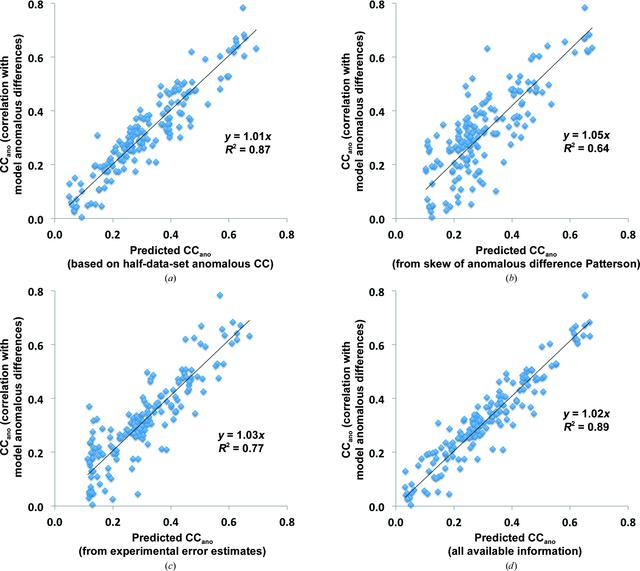disable zoom     view article Figure 5 Cross-validation of Bayesian estimates of useful anomalous correlation. The Bayesian estimator described in §2.3.1 was used to generate estimates of the useful anomalous correlation based on (a) the half-data-set anomalous correlation, (b) the skew of the anomalous difference Patterson, (c) the normalized error in the anomalous differences and (d) all three. The data used are from the 166 SAD data sets in Fig. 2where unmerged data were available so that all three measures were available. To carry out the cross-validation the data set to be analysed was left out of the training set for the estimator. The x coordinate for each point is the value of the useful anomalous correlation from the Bayesian estimator for one SAD data set and the y coordinate is the actual value of the useful anomalous correlation for that data set.STRUCTURALBIOLOGY
ISSN: 2059-7983
Volume 72| Part 3| March 2016| Pages 359-374